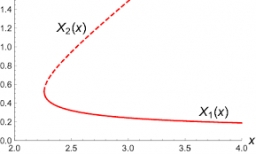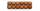# Simple equation 9

Solve the following equation: -8y+5=-9y+9

y =  4

### Step-by-step explanation:

-8y+5=-9y+9

-8•y+5=-9•y+9

y = 4

Our simple equation calculator calculates it.Did you find an error or inaccuracy? Feel free to write us. Thank you!Tips to related online calculators
Do you have a linear equation or system of equations and looking for its solution? Or do you have a quadratic equation?

## Related math problems and questions:

• Equation 11Solve equation: 0=y-1,2.(y-1,5)
• Simple equationSolve the following simple equation: 2. (4x + 3) = 2-5. (1-x)
• Two equationsSolve equations (use adding and subtracting of linear equations): -4x+11y=5 6x-11y=-5
• Substitutionsolve equations by substitution: x+y= 11 y=5x-25
• Simple equation 8Solve the following equation: 36=-(1+7x)-6(-7-x)
• Simple equationsSolve system of equations: 5x+3y=5 5x+7y=25
• Simple equation 2Find X in this simple equation: X/9 = 96/108
• Equation 25Solve following simple equation: 3/4(x+5)=1/2(x+9)
• Equation 29Solve next equation: 2 ( 2x + 3 ) = 8 ( 1 - x) -5 ( x -2 )
• Consider 2Consider the following formula: y = 3 ( x + 5 ) ( x - 2 ) Which of the following formulas is equivalent to this one? A. Y=3x2+9x-30 B. Y=x2+3x-10 C. Y=3x2+3x-10 D. Y=3x2+3x-30
• Simple equation 324 = n • 27, solve for n
• Count of rootsHow many solutions has equation x. y = 7757 with two unknowns on the set of natural numbers?
• EquationSolve equation and check the result: 1.4x - 3/2 + x - 9,8 = x + 0,4/3 - 7 + 1,6/6
• Find xSolve: if 2(x-1)=14, then x= (solve an equation with one unknown)
• Simplify:Simplify: 8(−9 − 5x) + 4b use x = 9 and b = −9
• EquationsSolve following system of equations: 6(x+7)+4(y-5)=12 2(x+y)-3(-2x+4y)=-44
• Equation with xSolve the following equation: 2x- (8x + 1) - (x + 2) / 5 = 9